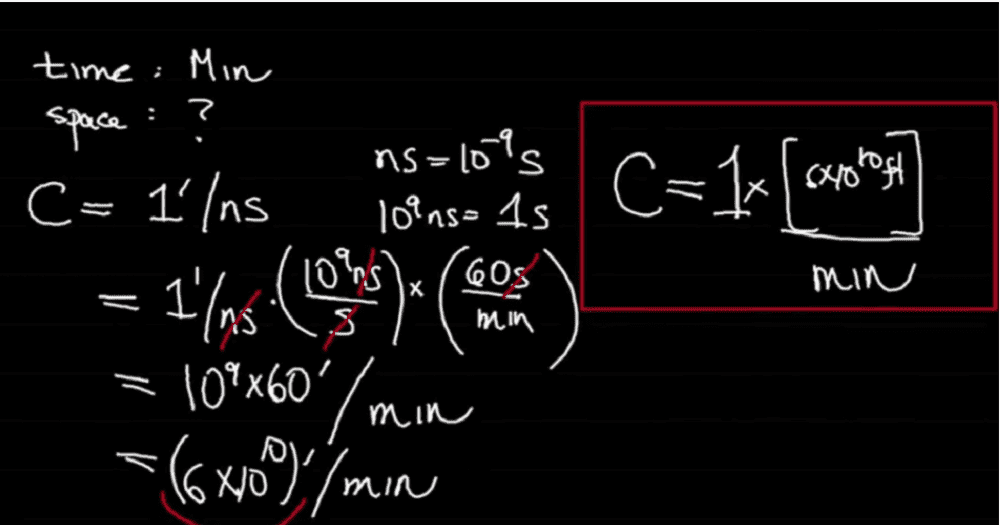# Dimensional Analysis and units of time

gtguhoij
Homework Statement:
If someone is using the minute as their unit of time, what unit should they choose for length so the speed of light will be one in those units?
Relevant Equations:
Dimensional Analysis
time = x(min)
distance = 1(y)
y = unknown units

I think the answer should be 1(y)/ Min. This is not correct becase 1(y) is unknown. Any help?

I have the answer but am confusedHomework Helper
Gold Member
2022 Award
How far does light travel in one minute?

gtguhoij
A light minute.

Homework Helper
Gold Member
2022 Award
A light minute.
Is that a unit of distance, then?

gtguhoij
I think so but from your question it seems it is not. Why is it not?
Do you mean something like 17,987,547,480.00 meters/minutes? except it is = 1 y/minutes?
Where y is an unknown unit of distance.
But how do I make y/minutes = 1 for velocity of light?

I am sorry I am just confused.

Homework Helper
Gold Member
2022 Award
I think so but from your question it seems it is not. Why is it not?
A question is a question. A question is not a statement.

Is a light minute a unit of distance?

gtguhoij
Yes

Homework Helper
Gold Member
2022 Award
Yes
And if you measure distance in light minutes and time in minutes, what is the numerical value of the speed of light in those units?

•Steve4Physics
Homework Helper
Gold Member
2022 Award
1 light minute.
That has the wrong dimensions for speed. Speed has dimensions of ##LT^{-1}##.

Gold Member
Let‘s try a different approach. You are trying to convert to a new unit of velocity (nuv) which has magnitude 1. It is given that the new unit of time (nut) is 1 minute. You are trying to figure out the new unit of distance (nud). In these units, velocity is measured in nud’s per minute. The question is how many ft are in a nud so that when you convert the speed of light from ft/ns to nud’s/min you get 1 nud/min.

•Steve4Physics and gtguhoij
gtguhoij
And if you measure distance in light minutes and time in minutes, what is the numerical value of the speed of light in those units?

Whoops I calculated time and velocity.

##d = vt##
##t = d/v = ##

##v = \frac {186 000 miles }{second} * \frac {60 sec}{1 min} = \frac{111 600 000 mile} {min} ##

## t = \frac {1L ~Min} {11 160 000 ~miles~min} = ##
##\frac {1c} {1116000~miles~min } =## ##\frac {186 000~ miles} {11160000miles~min } = 0.0166 min##
My one question is shouldn't it be ## \frac {0.0166} {min}##. How do I move the minutes units to the top? I am pretty sure there is an algbra rule I just can't remember it. I am pretty sure it is different rule then what I used in the first part of ## t = ... ##

I referenced this site rule number 3 for the first step in ##t=...## https://algebrarules.com/.

If I made any mistakes let me know.

Last edited:
Homework Helper
Gold Member
2022 Award
Whoops I calculated time and velocity.

##d = vt##
##t = d/v = ##

##v = \frac {186 000 miles }{second} * \frac {60 sec}{1 min} = \frac{111 600 000 mile} {min} ##

## t = \frac {1L ~Min} {11 160 000 ~miles~min} = ##
##\frac {1c} {1116000~miles~min } =## ##\frac {186 000~ miles} {11160000miles~min } = 0.0166 min##
My one question is shouldn't it be ## \frac {0.0166} {min}##. How do I move the minutes units to the top? I am pretty sure there is an algbra rule I just can't remember it. I am pretty sure it is different rule then what I used in the first part of ## t = ... ##

I referenced this site rule number 3 for the first step in ##t=...## https://algebrarules.com/.

If I made any mistakes let me know.
I'm lost for words. I have no idea what you are trying to do: ##c = 1## light-minute per minute, by definition.

Homework Helper
Gold Member
2022 Award
This might help...

You do not need to use c = 186,000 miles/second.

An apostrophe is the symbol for 1 foot.
It appears you are told that c = 1’/ns. This means c = 1ft/ns.
So light moves 1 foot each nanosecond (approximately true in a vacuum).

How far (in feet) does light travel in 1 minute?

This distance is the size of the required unit (which you call ‘y’), because light travels a distance ‘y’ in 1 minute. So speed = 1 y/min.

The question simply wants the value of y expressed in feet.

Homework Helper
2022 Award
I'm lost for words. I have no idea what you are trying to do: ##c = 1## light-minute per minute, by definition.

And in terms of other units: 1 light minute is 60 light seconds. A light second is 299 792 458 meters by definition.

Therefore a light minute is $60 \times 299\,792\,458$ meters.

gtguhoij
I get what to do now to get the correct answer. Is the math correct in post #11? Even though it doesn't answer the question.

Gold Member
I get what to do now to get the correct answer. Is the math correct in post #11? Even though it doesn't answer the question.
No. One light minute divided by the speed of light equals 1 minute. There’s a typo in the velocity equation (1 too many zeros). In the time equation, the units of velocity in the denominator are wrong and there are issues with your calculated value of a light minute (it looks like you are mixing different time units, seconds and minutes).

Last edited:
•gtguhoij